# Generating Cats Using Generative Adversarial Networks and Principal Component Analysis## Aim

This article will provide you with a general framework of a Generative Adversarial Network written using the Keras library. You will be able to use this generic GAN template to train generative models using your own image datasets.

The complete version of the code is available at this Github repository .

## Dataset

The dataset I used for this project can be downloaded from Kaggle. I used a dataset of 64x64 cat pictures to train the model. This dataset is particularly easy to work with for GANs because of how little variance there is between the images. Other datasets with wildly varying images can make it harder for your Generative Adversarial Network to converge.

In this supervised learning task, these cat images are the Y values in our dataset. The images are what our generative models will output. The question is now what we will use as our X values to map as input to our images. We could just map these images to a random distribution of noise. This rudimentary technique works for certain datasets. This GIF below shows sampling through interpolated points in the latent space of a generative model.This model was trained using a Gaussian distribution of noise as input, and this same dataset as output. The model struggled to converge given the randomness of the input and produced mediocre results with non expressive outputs.

Depending on the dataset, using random inputs mapped to images can be good enough for certain GANs to converge. The classic MNIST benchmark dataset is simple enough to work with random inputs. This Interactive GAN was also trained using a Gaussian distribution of noise mapped to a very low variance dataset.

But ultimately, the problem with this technique is that it is hard for Neural Networks to recreate images from the dataset using completely random numbers as input. Ideally, the X values of our dataset would be some meaningful representation of the Y value images in our dataset. This would allow the Generator model to find meaningful detail in the input to upsample and accurately recreate the output. The question now is, how do we generate meaningful X values to go with each Y value image in our dataset?

This brings us to an unsupervised learning technique called Principal Component Analysis (PCA). PCA allows us to project data onto a lower dimension by projecting the data onto the Eigenvectors of the covariance matrix of the dataset. Using this technique, we are able to project each 64x64x3 image in our dataset to a 512 element vector. This is equivalent to using 4.16% of the space to store the data while retaining 95.3% of the information. This is how we are going to get a meaningful input vector for each output image we have in our dataset. The code behind processing this data will be fully explained in the load_data() method. load_data() method.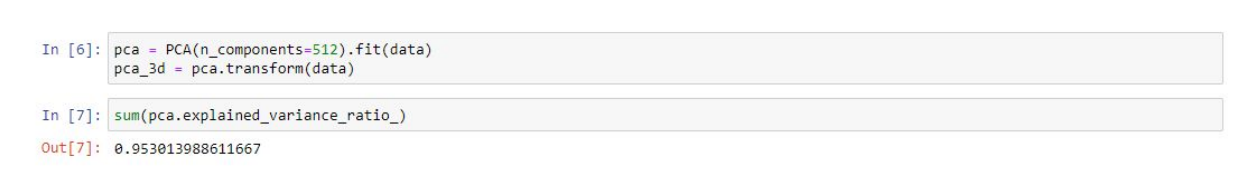## Imports

In :
```from keras.layers import Input, Dense, Reshape, Flatten
from keras.layers.convolutional import UpSampling2D, Conv2D, MaxPooling2D
from keras.models import Sequential, Model
from sklearn.utils import shuffle
from sklearn.decomposition import PCA
import matplotlib.pyplot as plt
import numpy as np
import os
from PIL import Image
```

`Using TensorFlow backend`
This project requires the following libraries;
• Keras (I use 2.3.1)
• Tensorflow (I use 1.14.0)
• Sklearn
• Scipy
• Numpy
• Matplotlib
• PIL
• Keract (Optional, for Model Visualization)

## Set Parameters

To start, we will define parameters regarding local file paths and settings of the Neural Networks. The current parameters are designed to be used on a GPU with 8GBs of VRAM. If you are working with a less powerful GPU, then reduce the number of convolutional filters and kernel size accordingly. The batch size can also be reduced for memory constraints. If you want to use your own dataset, adjust the data_path to point to the folder containing the images. If the images are PNGs, then set the png Boolean variable to True. This will remove the alpha layer from the images during data preprocessing in load_data() .

In :
```# Folder containing dataset

# Dimensions of the images inside the dataset
img_dimensions = (64,64,3)

# Folder where you want to save to model as well as generated samples
Set the dataset_path = r"C:\Users\Vee\Desktop\python\GAN\pca_new"

# How many epochs between saving your model
interval = 10

# How many epochs to run the model
epoch = 500

# How many images to train at one time.
# Ideally this number would be a factor of the size of your dataset
batch = 181

# How many convolutional filters for each convolutional layer of the generator and the discrminator
conv_filters = 64

# Size of kernel used in the convolutional layers
kernel = (5,5)

# Boolean flag, set to True if the data has pngs to remove alpha layer from images
png = False```

## Create Deep Convolutional GAN Class

This class contains 8 methods.
• __init __ (self): The class is initialized by defining the dimensions of the input vector as well as the output image. The Generator and Discriminator models get initialized using build_generator() and build_discriminator() .
• build_generator(self): Defines Generator model. There are 5 convolutional layers, upsampling from 8x8x8 to 64x64x3. Gets called when the DCGAN class is initialized.
• build_discriminator(self): Defines Discriminator model. There are 5 convolutional layers, downsampling from 64x64x3 to 1 scalar prediction. Gets called when the DCGAN class is initialized.
• load_data(self): Loads data from user specified file path, data_path Uses PCA to project the image dataset onto a lower dimension as X_Train dataset. Processes image dataset and reshape to 4 dimensions for Y_Train dataset. Gets called in the train() method.
• train(self, epochs, batch_size, save_interval): Trains the Generative Adversarial Network. Each epoch trains the model using the entire dataset split up into chunks defined by batch_size If the epoch is at save_intervalthen the method calls save_imgs() to generate samples and saves the model of the current epoch.
• save_imgs (self, epoch, gen_imgs, y_points): Saves the model and generates prediction samples for a given epoch at the user specified path, Set the dataset_pathEach sample contains 8 generated predictions and 8 training samples.

## Initialization

In :
```class DCGAN():

# Initialize parameters, generator, and discriminator models
def __init__(self):

# Set dimensions of the output image
self.img_rows = img_dimensions
self.img_cols = img_dimensions
self.channels = img_dimensions
self.img_shape = (self.img_rows, self.img_cols, self.channels)

# Set dimensions of the input noise
self.latent_dim = 512

# Chose optimizer for the models

# Build and compile the discriminator
self.discriminator = self.build_discriminator()
self.discriminator.compile(loss='binary_crossentropy',
optimizer=optimizer,
metrics=['accuracy'])

# Build the generator
self.generator = self.build_generator()
generator = self.generator

# The generator takes noise as input and generates imgs
z = Input(shape=(self.latent_dim,))
img = self.generator(z)

# For the combined model we will only train the generator
self.discriminator.trainable = False

# The discriminator takes generated images as input and determines validity
valid = self.discriminator(img)

# The combined model  (stacked generator and discriminator)
# Trains the generator to fool the discriminator
self.combined = Model(z, valid)
self.combined.compile(loss='binary_crossentropy', optimizer=optimizer)```

When the DCGAN class is initialized, we define the size of the images the Neural Network should expect from the dataset. This is specified by the Tuple img_dimensions We also define the latent dimensions, which is the size of our input vector generated from PCA. This is specified by the latent_dim integer.

The optimizer we are using for both models is the Adam optimizer. Feel free to experiment with the learning rate and beta values of the optimizer and see what kind of results you get.

The architecture of the Generative Adversarial Network is defined here, with both models using Binary Cross Entropy loss. The choice of Binary Cross Entropy as the loss function is explained here Feel free to experiment with other loss functions but just keep in mind that both models must use the same loss function.

In :
```class DCGAN():

# Initialize parameters, generator, and discriminator models
def __init__(self):

# Set dimensions of the output image
self.img_rows = img_dimensions
self.img_cols = img_dimensions
self.channels = img_dimensions
self.img_shape = (self.img_rows, self.img_cols, self.channels)

# Set dimensions of the input noise
self.latent_dim = 512

# Chose optimizer for the models

# Build and compile the discriminator
self.discriminator = self.build_discriminator()
self.discriminator.compile(loss='binary_crossentropy',
optimizer=optimizer,
metrics=['accuracy'])

# Build the generator
self.generator = self.build_generator()
generator = self.generator

# The generator takes noise as input and generates imgs
z = Input(shape=(self.latent_dim,))
img = self.generator(z)

# For the combined model we will only train the generator
self.discriminator.trainable = False

# The discriminator takes generated images as input and determines validity
valid = self.discriminator(img)

# The combined model  (stacked generator and discriminator)
# Trains the generator to fool the discriminator
self.combined = Model(z, valid)
self.combined.compile(loss='binary_crossentropy', optimizer=optimizer)```

The first method we are adding to the DCGAN class is load_data() This will preprocess all images within the user specified path, data_path . This method gets called inside the train() method to load the data before training.

This is where the dataset gets projected onto a lower dimensional space using PCA. The Sklearn PCA method requires the data passed in to be 2 dimensions, so we are squishing the 64x64x3 images to be a 12288 vector. We are also normalizing the data to be between 0 and 1. RGB pixel values range from 0 to 255, so we are dividing the reshaped vector by 255.

Lastly, we shuffle the train() method datasets before returning the two arrays. I wrote the train() method to train the models on the dataset sequentially, incrementing by the batch size each iteration. So it is important to shuffle the dataset to not introduce weird biases relating to the way the dataset is sequentially ordered train() .

## Build Generator

``` # Define Generator model. There are 5 convolutional filters, upsampling from (8x8x8) to (64x64x3)
def build_generator(self):

model = Sequential()

# Input Layer
model.add(Dense(8 * 8 * 8, input_dim=self.latent_dim))

# 1st Convolutional Layer

# Upsample the data (8x8 to 16x16)

# 2nd Convolutional Layer

# Upsample the data (16x16 to 32x32)

# 3rd Convolutional Layer

# Upsample the data (32x32 to 64x64)

# 4th Convolutional Layer

# 5th Convolutional Layer (Output Layer)
model.summary()

noise = Input(shape=(self.latent_dim,))
img = model(noise)

return Model(noise, img)
```

The second method we are adding to the DCGAN class is build_generator(). This method is called when the class is first initialized. The architecture of the Generator model is designed here. The model summary will give you a clearer idea on what is actually happening inside this model.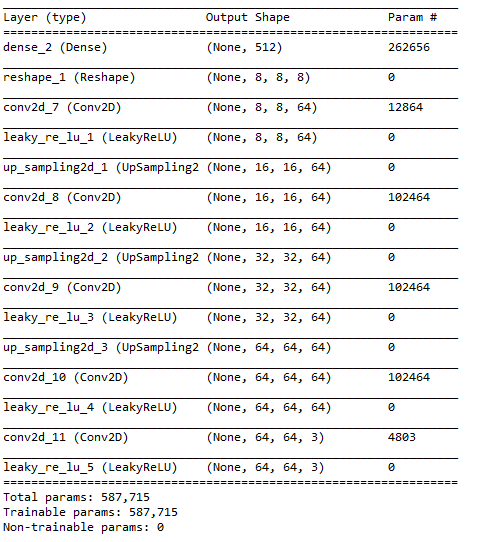The input of the Generator model is a vector of 512 numbers. The vector is then reshaped to an 8x8x8 tensor. This tensor is then upsampled to 16x16, 32x32, and finally 64x64. The output Convolutional layer contains 3 filters representing the Red, Green, and Blue channels of an RGB image respectively.

## Build Discriminator

```    The third method we are adding to the DCGAN class is
def build_discriminator(self):

model = Sequential()

# Input Layer

# Downsample the data (64x64 to 32x32)

# 1st Convolutional Layer

# Downsample the data (32x32 to 16x16)

# 2nd Convolutional Layer

# Downsample the data (16x16 to 8x8)

# 3rd Convolutional Layer

# 4th Convolutional Layer

# 5th Convolutional Layer

# Output Layer

model.summary()

img = Input(shape=self.img_shape)
validity = model(img)

return Model(img, validity)```

The third method we are adding to the DCGAN class is build_discriminator() . This method is called when the class is first initialized. The architecture of the Discriminator model is designed here. The model summary will give you a clearer idea on what is actually happening inside this model.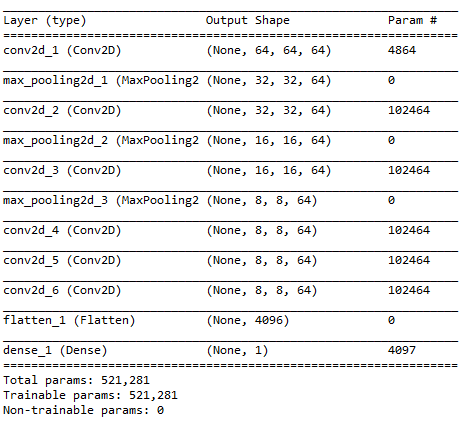The input of the Discriminator model is a 64x64x3 tensor. The tensor is then downsampled to 32x32, 16x16, and 8x8. This 8x8 tensor is then flattened and passed to the output layer. The final dense layer outputs a single scalar number, representing the prediction of the discriminator model. This prediction represents the confidence of the model in determining if the input image is “real”. A prediction of 1 means the model thinks that the image is from the original dataset. A prediction of 0 means that the model thinks that the image was generated by the Generator model.

## Train

``` # Train the Generative Adversarial Network
def train(self, epochs, batch_size, save_interval):

# Prevent script from crashing from bad user input
if(epochs <= 0):
epochs = 1

if(batch_size <= 0):
batch_size = 1

# Normalizing data to be between 0 and 1
Y_train = Y_train/255

valid = np.ones((batch_size, 1))
fake = np.zeros((batch_size, 1))

# Placeholder arrays for Loss function values
g_loss_epochs = np.zeros((epochs, 1))
d_loss_epochs = np.zeros((epochs, 1))

# Training the GAN
for epoch in range(1, epochs + 1):

# Initialize indexes for training data
start = 0
end = start + batch_size

# Array to sum up all loss function values
discriminator_loss_real = []
discriminator_loss_fake = []
generator_loss = []

# Iterate through dataset training one batch at a time
for i in range(int(len(X_train)/batch_size)):

# Get batch of images
imgs = Y_train[start:end]
noise = X_train[start:end]

# ---------------------
#  Train Discriminator
# ---------------------

# Make predictions on current batch using generator
gen_imgs = self.generator.predict(noise)

# Train the discriminator (real classified as ones and generated as zero)
d_loss_real = self.discriminator.train_on_batch(imgs, valid)
d_loss_fake = self.discriminator.train_on_batch(gen_imgs, fake)
d_loss = 0.5 * np.add(d_loss_real, d_loss_fake)

# ---------------------
#  Train Generator
# ---------------------

# Train the generator (wants discriminator to mistake images as real)
g_loss = self.combined.train_on_batch(noise, valid)

# Add loss for current batch to sum over entire epoch
discriminator_loss_real.append(d_loss)
discriminator_loss_fake.append(d_loss)
generator_loss.append(g_loss)

# Increment image indexes
start = start + batch_size
end = end + batch_size

# Get average loss over the entire epoch
loss_data = [np.average(discriminator_loss_real),np.average(discriminator_loss_fake),np.average(generator_loss)]

#save loss history
g_loss_epochs[epoch - 1] = loss_data

# Average loss of real data classification and fake data accuracy
d_loss_epochs[epoch - 1] = (loss_data + (1 - loss_data)) / 2

# Print average loss over current epoch
print ("%d [D loss: %f, acc.: %.2f%%] [G loss: %f]" % (epoch, loss_data, loss_data*100, loss_data))

# If epoch is at intervale, save model and generate image samples
if epoch % save_interval == 0:

# Select 8 random indexes
idx = np.random.randint(0, X_train.shape, 8)
# Get batch of generated images and training images
x_points = X_train[idx]
y_points = Y_train[idx]

# Plot the predictions next to the training imgaes
self.save_imgs(epoch, self.generator.predict(x_points), y_points)

return g_loss_epochs, d_loss_epochs

# Save the model and generate prediction samples for a given epoch
def save_imgs(self, epoch, gen_imgs, y_points):

# Define number of columns and rows
r, c = 4, 4

# Placeholder array for MatPlotLib Figure Subplots
subplots = []

# Unnormalize data to be between 0 and 255 for RGB image
gen_imgs = np.array(gen_imgs) * 255
gen_imgs = gen_imgs.astype(int)
y_points = np.array(y_points) * 255
y_points = y_points.astype(int)

# Create figure with title
fig = plt.figure(figsize= (40, 40))
fig.suptitle("Epoch: " + str(epoch), fontsize=65)

# Initialize counters needed to track indexes across multiple arrays
img_count = 0;
index_count = 0;
y_count = 0;

# Loop through columns and rows of the figure
for i in range(1, c+1):
for j in range(1, r+1):
# If row is even, plot the predictions
if(j % 2 == 0):
img = gen_imgs[index_count]
index_count = index_count + 1
# If row is odd, plot the training image
else:
img = y_points[y_count]
y_count = y_count + 1
plt.imshow(img)
img_count = img_count + 1

# Add title to columns of figure
subplots.set_title("Training", fontsize=45)
subplots.set_title("Predicted", fontsize=45)
subplots.set_title("Training", fontsize=45)
subplots.set_title("Predicted", fontsize=45)

# Save figure to .png image in specified folder
fig.savefig(Set the dataset_path + "\\epoch_%d.png" % epoch)
plt.close()

# save model to .h5 file in specified folder
self.generator.save(Set the dataset_path + "\\generator" + str(epoch) + ".h5")```

The fourth method we are adding to the DCGAN class is train(). This method will train the network for a specified number of epochs in increments specified by the batch size. When the training completes, the method will return two arrays representing the loss values of both models across every epoch. The loss values can be plotted using Matplotlib.

You should track the loss values and stop training the network if it starts collapsing. The network collapses if one of the models gets close to 0 loss.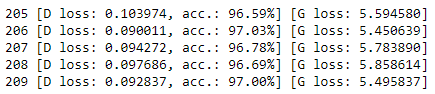If the Generator gets close to 0 loss, then that means the Generator has figured out how to make an image that will fool the discriminator everytime. This will usually result in the Generator only being able to produce one type of image, also known as mode collapse .

If the Discriminator gets close to 0 loss, then that means that the Discriminator has figured out how to distinguish between the training data and generated images very accurately. This will cause the Generator to be unable to continue to learn from the discriminator, also known as the vanishing gradient problem .

To avoid losing our progress when our network collapses, we will save the model every few epochs. The user defined parameter, interval, will determine how often the model gets saved. Every time the current epoch lands on the defined interval, save_imgs() gets called. The method will save an image of some predicted samples to get a snapshot of how good the model was during that epoch.

## Save Images

```    # Save the model and generate prediction samples for a given epoch
def save_imgs(self, epoch, gen_imgs, y_points):

# Define number of columns and rows
r, c = 4, 4

# Placeholder array for MatPlotLib Figure Subplots
subplots = []

# Unnormalize data to be between 0 and 255 for RGB image
gen_imgs = np.array(gen_imgs) * 255
gen_imgs = gen_imgs.astype(int)
y_points = np.array(y_points) * 255
y_points = y_points.astype(int)

# Create figure with title
fig = plt.figure(figsize= (40, 40))
fig.suptitle("Epoch: " + str(epoch), fontsize=65)

# Initialize counters needed to track indexes across multiple arrays
img_count = 0;
index_count = 0;
y_count = 0;

# Loop through columns and rows of the figure
for i in range(1, c+1):
for j in range(1, r+1):
# If row is even, plot the predictions
if(j % 2 == 0):
img = gen_imgs[index_count]
index_count = index_count + 1
# If row is odd, plot the training image
else:
img = y_points[y_count]
y_count = y_count + 1
plt.imshow(img)
img_count = img_count + 1

# Add title to columns of figure
subplots.set_title("Training", fontsize=45)
subplots.set_title("Predicted", fontsize=45)
subplots.set_title("Training", fontsize=45)
subplots.set_title("Predicted", fontsize=45)

# Save figure to .png image in specified folder
fig.savefig(Set the dataset_path + "\\epoch_%d.png" % epoch)
plt.close()

# save model to .h5 file in specified folder
self.generator.save(Set the dataset_path + "\\generator" + str(epoch) + ".h5")```

The fifth and last method we are adding to the DCGAN class is save_imgs() . This method will save the model at the current epoch and plot 8 training images as well as their predicted values.This method is currently configured to save every 5 epochs. This can be adjusted with the parameter, interval. Frequently saving your model is a good way to track the progress your network is making during the training process.

## Initializing The DCGAN Class

We are now done with creating the DCGAN class and ready to train our Generative Adversarial Network. First, we need to create an instance of the class and assign it to a variable.

In :

`dcgan = DCGAN()`

This will initialize the Generator and Discriminator models and print their summaries.

## Training The Generative Adversarial Network

Now that we have our DCGAN class object, we just need to call the train() method to start training. With this script, you should generally pick a high number of epochs for training and track the loss values throughout the process. If the network starts collapsing, then stop the training early and check the generated samples to figure out which model was the best performing one.

Train() method returns two arrays containing the loss values of the two models throughout training. We will assign these values to g_loss, and d_loss and plot them.

In :

`g_loss, d_loss = dcgan.train(epochs=epoch, batch_size=batch, save_interval=interval)`
```1 [D loss: 0.680393, acc.: 61.54%] [G loss: 0.732704]
2 [D loss: 0.654482, acc.: 60.98%] [G loss: 0.835647]
3 [D loss: 0.680507, acc.: 59.27%] [G loss: 0.832127]
4 [D loss: 0.667612, acc.: 61.23%] [G loss: 0.890128]```
`5 [D loss: 0.678923, acc.: 57.80%] [G loss: 0.878026]`
```6 [D loss: 0.669563, acc.: 59.07%] [G loss: 0.857507]
7 [D loss: 0.674293, acc.: 59.85%] [G loss: 0.880131]
8 [D loss: 0.667477, acc.: 58.76%] [G loss: 0.876913]
9 [D loss: 0.663820, acc.: 59.30%] [G loss: 0.891338]```
`10 [D loss: 0.659955, acc.: 59.45%] [G loss: 0.909291]`

## Plot Loss

In :
```plt.plot(g_loss)
plt.plot(d_loss)
plt.title('GAN Loss')
plt.ylabel('Loss')
plt.xlabel('Epoch')
plt.legend(['Generator', 'Discriminator'], loc='upper left')
plt.show()```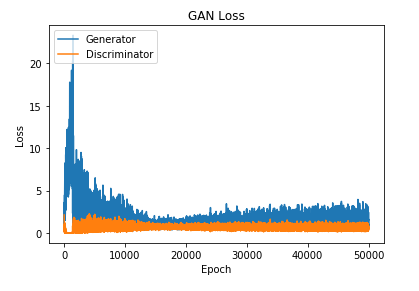## Conclusion

This article provides you with a general framework of training a Generative Adversarial Network using Keras. You will be able to create your own generative models with your own datasets using this script. The full version of this code can be found here. The script is currently configured for 64×64 images.If you want to train using datasets with images of a different size, you will need to adjust the img_dimensions parameter and adjust the amount UpSampling2D and MaxPooling2D layers accordingly.

Once you have trained a model you are satisfied with, you can use the PCA GAN Inference script to generate outputs and analyze your results. This script will also provide you with code to create GIFs of walking through the latent space of the Generator model such as the ones provided at the beginning of the article.

You can also use the Model Visualization script to get a look at what is happening inside each convolutional layer when your modelmakes a prediction.Researcher